## Thursday, June 12, 2008

### Derivation of the DOF Formulas

DOF limits
A symmetrical lens is illustrated at right. The subject, at distance s, is in focus at image distance v. Point objects at distances DF and DN would be in focus at image distances vF and vN, respectively; at image distance v, they are imaged as blur spots. The depth of field is controlled by the aperture stop diameter d; when the blur spot diameter is equal to the acceptable circle of confusion c, the near and far limits of DOF are at DN and DF. From similar triangles,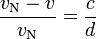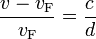It usually is more convenient to work with the lens f-number than the aperture diameter; the f-number N is related to the lens focal length f and the aperture diameter d bysubstituting into the previous equations and rearranging givesThe image distance v is related to an object distance s by the thin lens equationSubstituting into the two previous equations and rearranging gives the near and far limits of DOF: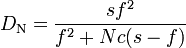### Hyperfocal distance

Setting the far limit of DOF DF to infinity and solving for the focus distance s giveswhere H is the hyperfocal distance. Setting the subject distance to the hyperfocal distance and solving for the near limit of DOF givesFor any practical value of H, the focal length is negligible in comparison, so that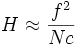Substituting the approximate expression for hyperfocal distance into the formulas for the near and far limits of DOF givesCombining, the depth of field DFDN is### Moderate-to-large distances

When the subject distance is large in comparison with the lens focal length,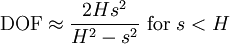For, the far limit of DOF is at infinity and the DOF is infinite; of course, only objects at or beyond the near limit of DOF will be recorded with acceptable sharpness.

### Close-up

When the subject distance s approaches the lens focal length, the focal length no longer is negligible, and the approximate formulas above cannot be used without introducing significant error. At close distances, the hyperfocal distance has little applicability, and it usually is more convenient to express DOF in terms of magnification. Substitutingand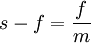into the formula for DOF and rearranging givesAt the hyperfocal distance, the terms in the denominator are equal, and the DOF is infinite. As the subject distance decreases, so does the second term in the denominator; when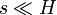, the second term becomes small in comparison with the first, and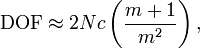so that for a given magnification, DOF is independent of focal length. Stated otherwise, for the same subject magnification, all focal lengths for a given image format give approximately the same DOF. This statement is true only when the subject distance is small in comparison with the hyperfocal distance, however. Multiplying the numerator and denominator of the exact formula by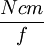givesDecreasing the focal length f increases the second term in the denominator, decreasing the denominator and increasing the value of the right-hand side, so that a shorter focal length gives greater DOF. The effect of focal length is greatest near the hyperfocal distance, and decreases as subject distance is decreased. However, the near/far perspective will differ for different focal lengths, so the difference in DOF may not be readily apparent. When the subject distance is small in comparison with the hyperfocal distance, the effect of focal length is negligible, and, as noted above, the DOF essentially is independent of focal length.

### Near : Far DOF ratio

From the “exact” equations for near and far limits of DOF, the DOF in front of the subject isand the DOF beyond the subject is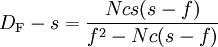The near:far DOF ratio is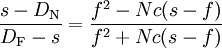This ratio is always less than unity; at moderate-to-large subject distances,, and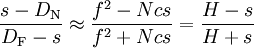When the subject is at the hyperfocal distance or beyond, the far DOF is infinite, and the near:far ratio is zero. It's commonly stated that approximately 1/3 of the DOF is in front of the subject and approximately 2/3 is beyond; however, this is true only when$s \approx H/3$.

At closer subject distances, it's often more convenient to express the DOF ratio in terms of the magnificationSubstitution into the “exact” equation for DOF ratio givesAs magnification increases, the near:far ratio approaches a limiting value of unity.

### Focus and f-number from DOF limits

Not all images require that sharpness extend to infinity; the equations for the DOF limits can be combined to eliminate Nc and solve for the subject distance. For given near and far DOF limits DN and DF, the subject distance is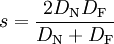The equations for DOF limits also can be combined to eliminate s and solve for the required f-number, giving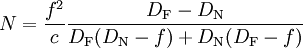When the subject distance is large in comparison with the lens focal length, this simplifies to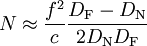Most discussions of DOF concentrate on the object side of the lens, but the formulas are simpler and the measurements usually easier to make on the image side. If vN and vF are the image distances that correspond to the near and far limits of DOF, the required f-number is minimum when the image distance v isThe required f-number is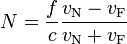The image distances are measured from the camera's image plane to the lens's image nodal plane, which is not always easy to locate. In most cases, focus and f-number can be determined with sufficient accuracy using the approximate formulaswhich require only the difference between the near and far image distances; focus is simply set to halfway between the near and far distances. View camera users often refer to the difference$v_{\mathrm N} \, - \, v_{\mathrm F}$ as the focus spread; it usually is measured on the bed or focusing rail. On manual-focus small- and medium-format lenses, the focus and f-number usually are determined using the lens DOF scales, which often are based on the two equations above.

For close-up photography, the f-number is more accurately determined using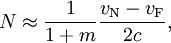where m is the magnification.

### Foreground and background blur

If the equation for the far limit of DOF is solved for c, and the far distance replaced by an arbitrary distance D, the blur disk diameter b at that distance isWhen the background is at the far limit of DOF, the blur disk diameter is equal to the circle of confusion c, and the blur is just imperceptible. The diameter of the background blur disk increases with the distance to the background. A similar relationship holds for the foreground; the general expression for a defocused object at distance D isFor a given scene, the distance between the subject and a foreground or background object is usually fixed; let that distance be represented bythenor, in terms of subject distance,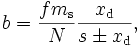with the minus sign used for foreground objects and the plus sign used for background objects. For a relatively distant background object,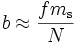In terms of subject magnification, the subject distance isso that, for a given f-number and subject magnification,Differentiating b with respect to f givesWith the plus sign, the derivative is everywhere positive, so that for a background object, the blur disk size increases with focal length. With the minus sign, the derivative is everywhere negative, so that for a foreground object, the blur disk size decreases with focal length.

The magnification of the defocused object also varies with focal length; the magnification of the defocused object is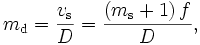where vs is the image distance of the subject. For a defocused object with some characteristic dimension y, the imaged size of that object isThe ratio of the blur disk size to the imaged size of that object then isso for a given defocused object, the ratio of the blur disk diameter to object size is independent of focal length, and depends only on the object size and its distance from the subject.

### Asymmetrical lenses

The discussion thus far has assumed a symmetrical lens for which the entrance and exit pupils coincide with the object and image nodal planes, and for which the pupil magnification is unity. Although this assumption usually is reasonable for large-format lenses, it often is invalid for medium- and small-format lenses.

For an asymmetrical lens, the DOF ahead of the subject distance and the DOF beyond the subject distance are given by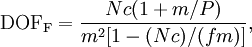where P is the pupil magnification.

Combining gives the total DOF: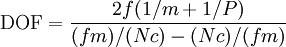When$s \ll H$, the second term in the denominator becomes small in comparison with the first, andWhen the pupil magnification is unity, the equations for asymmetrical lenses reduce to those given earlier for symmetrical lenses.

### Effect of lens asymmetry

Except for close-up and macro photography, the effect of lens asymmetry is minimal. A slight rearrangement of the last equation givesAs magnification decreases, the 1 / P term becomes smaller in comparison with the 1 / m term, and eventually the effect of pupil magnification becomes negligible.